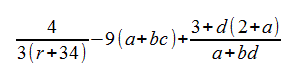# COP 2800 (Java Programming I) Homework Assignment #1

## Description:

Answer the following questions as briefly (but completely) as possible:

1. What is a source program?  What is a compiler?
2. What is the “JVM”?  What is the “JRE”?
3. What is an operating system?
4. Identify and fix the errors in the following code:
     public class Welcome  {
public void Main(String[] args) {
System.out.println('Welcome to Java!);
}
)
5. If a NoClassDefFoundError occurs when you attempt to run a Java program, what is the cause of the error?
6. Why doesn't the System class need to be imported?
7. Which of the following identifiers are valid?  Which are Java keywords? Applet, applet, a++, --a, 4#R, \$4, #44, apps, class, public, false, int, x, y, radius
8. What are the benefits of using constants?  How should you declare an int constant named SIZE with the value 20?
9. Assume that “int a=1” and “double d=1.0”, and that each expression is independent of the others.  What are the results of the following expressions (that is, the resulting value of a or d)?
    a = 46 / 9;
a = 46 % 9 + 4 * 4 - 2;
a = 45 + 43 % 5 * (23 * 3 % 2);
a %= 3 / a + 3;
d = 4 + d * d + 4;
d += 1.5 * 3 + (++a);
d -= 1.5 * 3 + a++;
10. If today is a Tuesday, what will be the day 100 days from now?  (Show the Java code to calculate that.)
11. Are the following statements syntactically correct?  If so, show the output.  If not, state the problem.
1. System.out.println("25 / 4 is " + 25 / 4);
2. System.out.println("25 / 4.0 is " + 25 / 4.0);
3. System.out.println("3 * 2 / 4 is " + 3 * 2 / 4 );
4. System.out.println("3.0 * 2 / 4 is " + 3.0 * 2 / 4);
12. How would you write the following arithmetic expression in Java?  (Note all variable names used are one-letter long.)

$\frac{4}{3\left(r+34\right)}-9\left(a+\mathit{bc}\right)+\frac{3+d\left(2+a\right)}{a+\mathit{bd}}$

      4                 3 + d(2+a)
-------  - 9(a+bc) + -----------
3(r+34)                 a+bd13. Evaluate the following two expressions:
      2 * 2 - 3 > 2 && 4 - 2 > 5

2 * 2 - 3 > 2 || 4 - 2 > 5
14. Write a Boolean expression that evaluates true if weight is greater than 50 pounds or height is greater than 60 inches.

## To be turned in:

Email your homework assignment, by copy-and-paste (no attachments please, to (homework submission).  If possible use the “text” and not the “HTML” mode of your email program.  Please use the subject similar to “Java Programming I Homework Assignment #1 Submission”, so I can tell which emails are submitted homework assignments.

Homework assignments will not be returned.  Please do not send as attachments.  Refer to the Homework and the Submitting Assignments sections of your syllabus for more information.

Confused?  Send questions about the homework assignment to (homework questions).  Please use a subject similar to “Java Programming I Homework Assignment #1 Questions” so I can tell which emails are questions about assignment quiz (and not submissions).